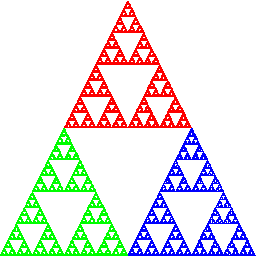## Rational Map AppletsThere are numerous applets on this page that may be used to investigate the dynamics of singularly perturbed maps in the complex plane.Degree three maps. This applet draws the Julia set and parameter plane for the function F(z) = z2 + c / z.Degree four maps. This applet draws the Julia set and parameter plane for the function F(z) = z2 + c / z2.Higher degree rational maps. This applet draws the Julia set and parameter plane for the function F(z) = zn + c / zm.Perturbations of the Douady rabbit. This applet draws the Julia set and parameter plane for the function F(z) = za -.12... + .75...i + c / z2, i.e., singular perturbations of the quadratic polynomial whose filled Julia set is the Douady rabbit.Perturbations of the basilica. This applet draws the Julia set and parameter plane for the function F(z) = z2 -1 + c / z2, i.e., singular perturbations of the quadratic polynomial whose filled Julia set is the basilica.Perturbations of the cubic rat (or mouse). This applet draws the Julia set and parameter plane for the function F(z) = z3 -i + c / z3, i.e., singular perturbations of the cubic polynomial whose filled Julia set is the rat.Sebastian's family. This applet draws the Julia set and parameter plane for the function F(z) = (z + C)n+d / zd which is semi-conjugate to the higher degree rational maps.Anti-holomorphic family. This applet is not quite ready yet. It will draw the Julia sets and parameter plane for functions that are anti-holomorphic singular perturbations.Perturbations of the airplane. This applet draws the Julia set and parameter plane for the function F(z) = z2 -1.75473 + c / z2, i.e., singular perturbations of the quadratic polynomial whose filled Julia set is the airplane.